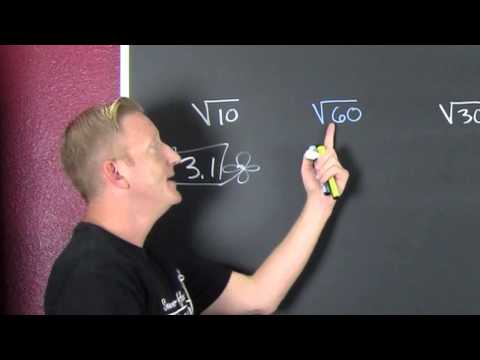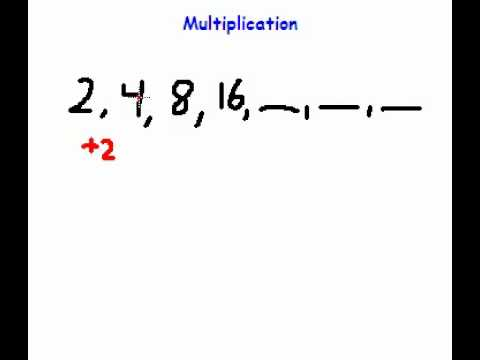## Root and Sequence Number

Subject: Compulsory Maths

#### Overview

A perfect square number is the product of two integers among the whole number. It is the perfect root of a particular number.A number that creates a specified number when it is multiplied by itself is said to be a square root.

#### Square and Square Root

Perfect Square Number
A perfect square number is the product of two integers among the whole number. It is the perfect root of a particular number. For example:
1× 1 = 12(1 squared) = 1 (1 is a perfect square number)
2× 2 = 22(2 squared) = 4 (4 is a perfect square number)
3× 3 = 32 (3 squared) = 9 (9 is a perfect square number)
Thus, a perfect square number is the product of two identical number 1, 4, 9, 16, 25, 36, 49, 64, 81, 100 . . . . . . . . . . . and so on.

Square Root

A number that creates a specified number when it is multiplied by itself is said to be a square root. It is the product of two equal number. The radical symbol ($\sqrt{}$) is used to denote square root of a number. For example:
$\sqrt{1}$ = 1
$\sqrt{4}$ = 2
$\sqrt{9}$ = 3
$\sqrt{64}$ = 8Note:
1 is the square number of 1, so 1 is called the square root of 1.
9 is the square number of 3, so 3 is called the square root of 9.
49 is the square number of 7, so 7 is called the square root of 49.

Factorization Method

In order to find out the square roots of a perfect square number, factorization method is used. For example:
Find the square root of 64 using factorization method.

So,
64 = 2× 2× 2× 2× 2× 2
= 22×22×22
∴ $\sqrt{64}$ = 2× 2× 2 = 8

Division Method

In order to find out the square roots of decimals and larger numbers, division method is used. For example:
Find the square root of 2704 using division method.

1. Starting from the unit place, pair up the numbers and use a bar mark for ease.
2. Take the first pair (i.e. 27) and think of the largest perfect square which is less or equal to 27 which is 25.
3. The square root of 25 is 5.
4. So, write 5 as both divisor and quotient. Multiply 5×5 and write just below 27, then subtract 25 from 27. You will get the remainder 2.
5. In divisor side add 5 + 5 = 10, which is the trial divisor.
6. Then, bring down the other pair i.e. 04. And the new dividend is 204.
7. 20 is two times divisible by 10, so write 2 in both quotient and divisor.
8. Here, the product of 102× 2 is 204. Write the result just below the dividend and subtract the result from the dividend. We get the remainder 0. Thus, 52 is the square root of 2704.

#### Cube and Cube Root

There is one unit cube so 1 is a cubic number.

There are 8 unit cubes. So, 8 is a cubic number.

There are 27 unit cubes, so 27 is a cubic number.

13 = 1× 1× 1 = 1 (1 is the cube of 1 & 1 is the cube root of 1)
23 = 2× 2× 2 = 8 (8 is the cube of 2 & 2 is the cube root of 8)
53 = 5× 5× 5 = 125(125 is the cube of 5 & 5 is the cube root of 125)
73 = 7 × 7 × 7 = 343 (343 is the cube of 7& 7is the cube root of 343)

Cube Number:Cubic number is the product of three iderntical number.
Cube root of a cubic number:Cube root of a cubic number is one of the identical numbers.It is denoted by the symbol $\sqrt{}$. For example:
or, $\sqrt{1}$ = 1
or,$\sqrt{27}$ = 3
or,$\sqrt{729}$ = 9 etc.

#### Sequence of Number

The list of number or object in a specific order is said to be a sequence. For example:

1. 1, 2, 3, 4, 5, 6, . . . . . . . . .
In this group of numbers, the common difference between each consecutive pair is 1. So, the numbers are in a fixed pattern.
2. 4, 8, 12, 16, 20, 24, . . . . . . . .
In this group of numbers, the second number of each consecutive pair is double to first one. Here, the number one in fixed pattern.

#### Rules for Sequence

Rule 1:
Lets supopose a sequence 2, 4, 6, 8, 10, 12, . . . . . .
Here, the common difference between each consecutive pair is 2. Consider 'n' as the number of terms of the sequence.
Then, the common difference is 2 and & the first term of the rule be 2n+ . . . . . . . . . . or 2n- . . . . . . . . . .
To get the first term 2,
n = 1
2n± . . . . . . .
= 2× 2 + 0 = 4
Similarly,
To get the second term 4,
n = 1
2n± . . . . . . .
= 2× 2 + 0 = 2
Here,
To get the nth term o0f the sequence, the rule must be 2n. In this way, we can find out the nth term of any sequence.

Rule 2:
Lets suppose the sequence 2, 5, 8, 11, 14, . . . . . . .
Consider that a denotes the first term and d denotes the common difference of the sequence.

 d = 5 - 2 = 3 d = 8 - 5 = 3 d = 11 - 8 = 3 d = 14 - 11 = 3 2 + 3 = 5 5 + 3 = 8 8 + 3 = 11 3 + 11 = 14

From the above illustration,
The 1st term (t1) = a = 2
The 2nd term (t2) = a + (2 - 1)d = a + d = a
The 3rd term (t3) = a + (3 - 1)d = a + 2d
The 4th term (t4) = a + (4 -1)d = a + 3d
∴ nth term (tn) = a + (n - 1)d
by using the nth term, we can find the 12th term of the sequence as :
8th term (t8) = a + (8 - 1)d
= a + 7d
= 2 + 7× 3
= 23

##### Things to remember
• A perfect square number is the product of two integers among the whole number.
• A number that creates a specified number when it is multiplied by itself is said to be a square root. It is the product of two equal number.
• The list of number or object in a specific order is said to be a sequence.
• It includes every relationship which established among the people.
• There can be more than one community in a society. Community smaller than society.
• It is a network of social relationships which cannot see or touched.
• common interests and common objectives are not necessary for society.
##### Videos for Root and Sequence Number##### Approximating a Square Root Without a Calculator##### Math Made Easy Solving Number Sequences

Solution:

144 ÷ 2 = 72 (remainder is 0)
72 ÷ 2 = 36 (remainder is 0)
36 ÷ 2 = 18 (remainder is 0)
18 ÷ 2 = 9 (renainder is 0)
9 ÷ 3 = 3 (remainder is 0)

Now,
144 = 2 × 2 × 2 × 2 × 3 × 3
144 = 22 × 22 × 3
∴ $\sqrt{144}$ = 2 × 2 × 3
= 12

Solution:

Square of 32 = 322
= 32 × 32
= 1024

Solution:

Square of 80 = 802
= 80 × 80
= 6400

Solution:

Square of 600 = 6002
= 600 × 600
= 360000

Solution:

576 ÷ 2 = 288 (remainder is 0)
288 ÷ 2 = 144 (remainder is 0)
144 ÷ 2 = 72 (remainder is 0)
72 ÷ 2 = 36 (remainder is 0)
36 ÷ 2 = 18 (remainder is 0)
18 ÷ 2 = 9 (remainder is 0)
9 ÷ 3 = 3 (remainder is 0)

Now,
576 = 2 × 2 × 2 × 2 × 2 × 2 × 3 × 3
576 = 22 × 22 × 22 × 32
∴ $\sqrt{576}$ = 2 × 2 × 2 × 3
= 24

Solution:

= $\sqrt{8}$ × 3$\sqrt{18}$ × 2$\sqrt{48}$
= $\sqrt{4 × 2}$ × 3$\sqrt{9 × 2}$ × 2$\sqrt{16 × 3}$
= 2$\sqrt{2}$ × 3 × 3$\sqrt{2}$ × 2 × 4$\sqrt{3}$
= 144$\sqrt{4}$
= 144$\sqrt{4 × 3}$
= 144 × 2$\sqrt{3}$
= 288$\sqrt{3}$

Solution:

When 10/10 children are arranged along length and breadth of a squared field the total number of children = 102
= 10 × 10
= 100
∴ The required additional number of children = 100 - 64
= 36

Solution:

1.96 = $\frac{196}{100}$
∴ $\sqrt{1.96}$ = $\sqrt{\frac{196}{100}}$
= $\frac{\sqrt{2 × 2 × 7 × 7}}{\sqrt{2 × 2 × 5 × 5}}$
= $\frac{\sqrt{2^2 × 7^2}}{\sqrt{2^2 × 5^2}}$
= $\frac{2 × 7}{2 × 5}$
= $\frac{14}{10}$
= 1.4

Solution:

= $\sqrt{\frac{256}{625}}$
= $\sqrt{\frac{2 × 2 × 2 × 2 × 2 × 2 × 2 × 2}{5 × 5 × 5 × 5}}$
= $\sqrt{\frac{2^2 × 2^2 × 2^2 × 2^2}{5^2 × 5^2}}$
= $\frac{2 × 2 × 2 × 2}{5 × 5}$
= $\frac{16}{25}$